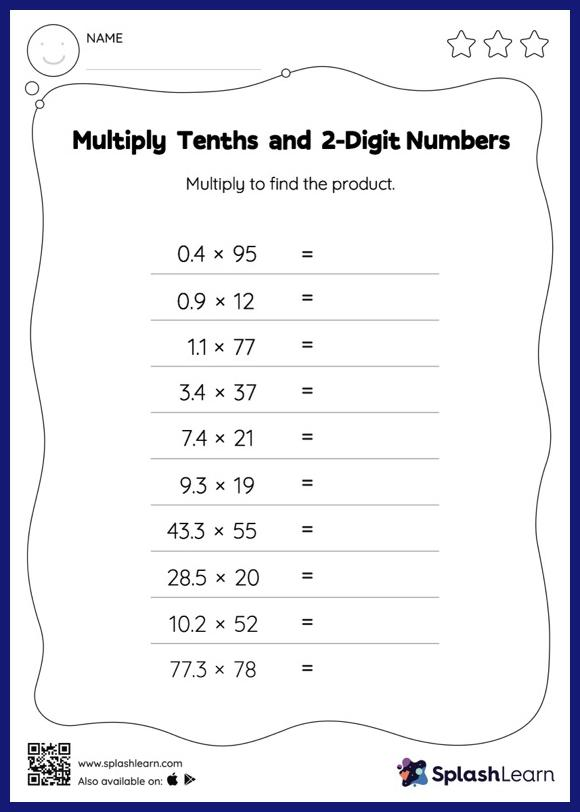# Multiply Tenths and 2-Digit Numbers: Horizontal Multiplication Worksheet

Home > Multiply Tenths and 2-Digit Numbers: Horizontal MultiplicationMultiply tenths and 2-digit numbers worksheet helps students develop proficiency with multiplication. This worksheet is about practicing with the horizontal format in which numbers are written side by side. To develop flexibility with numbers and operations, students need to have enough practice in this format and not just rely on the vertical/column method.